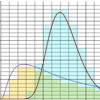#### You may also like### Understanding HypothesesCan you work out the means of these distributions using numerical methods?

# Stats Statements

##### Age 16 to 18Challenge Level

Russell from Willenhall School Sports College gave answers to five of the parts of this problem using a good mix of examples and results from distributions. Other contributions came from anonymous solution submitters and from teachers attending the Goldman Sachs Teacher Inspiration Day .

1) This doesn't have to be true. For example, in the set of results $0,0,58,72,51,63,60,56$ only $2$ out of $8$ got less than the average mark of $45$ because of the two extreme cases of the two people that put their name on the paper and then left! It is true if the results are normally (or symmetrically) distributed. The less symmetrical the distribution, the less likely that half the students will be under average.

This is usually true when lots of people take a test and the result is symmetrically distributed about the mean (like the normal distribution). It is not usually true when the results are skewed with large outliers for some reason

2) This is always false unless everyone gets exactly the same mark

3) Because the population is large, the question only says 'about half' and weights of adults are likely to be normally distributed, the result is likely to be true.

4) The total score over N games will be an even number. But the average might be even or odd. For example, scoring $10$ and $20$ over $2$ games gives an average of $15$. Scoring $10$, $20$ and $30$ over $3$ games gives an average of $20$.

5) This is sometimes true. For example, when rolling a fair die the standard deviation is $\sqrt{\frac{35}{12}} \approx 1.71$. I could roll the die three times and get $3, 4, 4$. This has a range of $1$, which is less than $1.71$. It can also obviously be false. For the example of the roll of a die you are very likely to observe a range larger than the standard deviation.

For a normal $N(0,1)$ distribution, the probability of a random variable $X$being within half a standard deviation of the mean is
$$P(-0.5< X< 0.5) = \Phi(0.5) -\Phi(-0.5) =0.69-0.31=0.38$$
The chance of 3 results occurring in this range is $0.388^3 = 0.05$. From this we can see that there is a small chance that 3 or more results will lie within 1 standard deviation of each other. (although this does not show it directly, because we could in a very unlikely set of results draw 3 numbers far from the mean which just happen to be close to each other)

We think that this helps to show that in almost all situations it is very unlikely that 3 or more randomly generated numbers are within 1 standard deviation of each other.

6) This is definitely true for distributions like normal where the range of possible values is infinite. Let's look at a different distribution. For a binomial distribution $B(N, p)$ the variance is $Np(1-p)$. With a binomial distribution the smallest possible outcome is $0$ and the largest is $N$. So the theoretical maximum range is $N$. The result is true for a binomial $B(N, p)$ if
$$\sqrt{Np(1-p)}\leq \frac{1}{2}N$$
This is only true in the case that $p(1-p)\leq \frac{N}{4}$ which is only false in the special case when $N=1$ and $p=0.5$. For a dice, half the range is 3 which is bigger then the standard deviation of $1.8$. So it seems that the result can be false, but only under very special circumstances.

7) Chebyshev's inequality says that the probability that a random number is more then $k$ standard deviations from the mean is not more than $\frac{1}{k^2}$. So, in this case the probability would be $\frac{1}{9}$. This means that the result is sometimes false. For the special case of a normal distribution, the chance of being within $3$ standard deviations of the mean is $0.0027$. So, the result is true for normal distributions.

8) This is always true by the law of large numbers, assuming that the average outcome is defined.  (The precise statement of the law of large numbers is somewhat technical, but in most everyday cases this is true.)

9) This is always the case, using Chebyshev's inequality. For a normal distribution, the probability of being within 10 standard deviations is about $1.5\times 10^{-23}$. So, for most distributions it is really, really, really likely that the sample is within 10 standard deviations of the mean.

10) Although this sounds like it ought to be true, it is not. This counter example shows why. The correlation between two random variables $X$ and $Y$ with standard deviations $\sigma_X$ and $\sigma_Y$ is
$$\frac{E(XY)-E(X)E(Y)}{\sigma_X\sigma_Y}$$
So, this is zero if and only if $E(XY) = E(X)E(Y)$.
Consider rolling a die twice. Let $A$ and $B$ be the result in each case. The make two new random variables $X=A+B$ and $Y=A-B$. Then $E(XY) = E((A+B)(A-B)) = E(A^2-B^2) = E(A^2) - E(B^2)$. Since $A$ and $B$ are identically distributed, we see that $E(XY)=0$. Also, it is easy to see that $E(Y)=0$. So, the two random variables $X$ and $Y$ have correlation zero. However, they are clearly dependent

So we have shown that correlation zero does not imply independence, although independence zero DOES imply zero correlation.